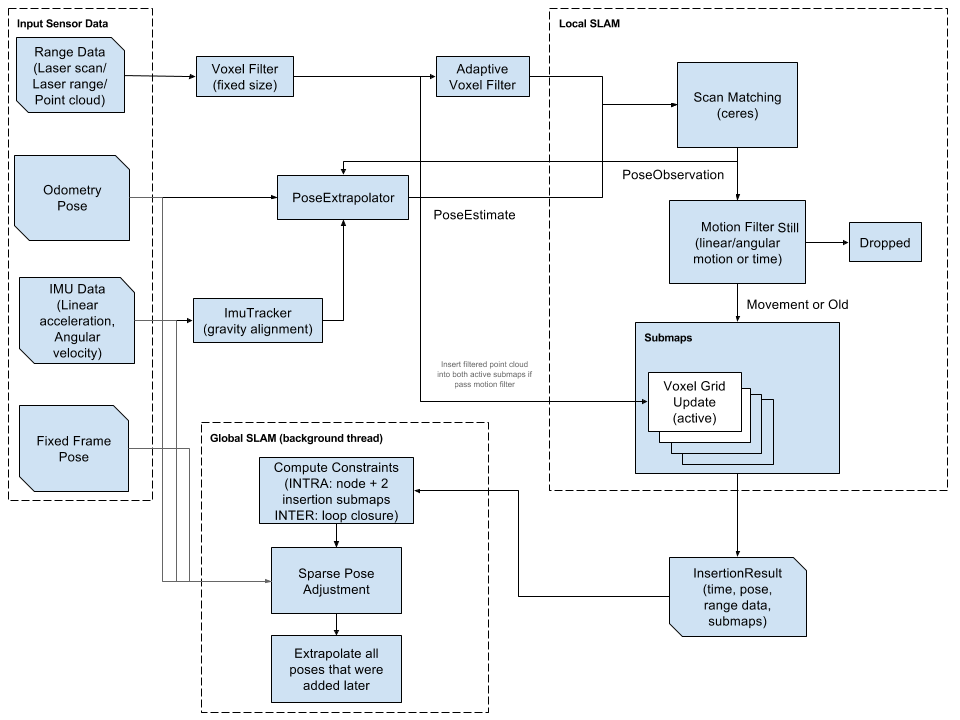## Tuesday, June 5, 2012

### Let's Get Moving...

This is a video of my robot taking infrared commands from an IHome remote that I rummaged from a pile of my junk.  I programmed the little guy in C and the code can be seen below.  I had to calibrate the motors to run at the same speed and they often don't go perfectly together.  If I used encoders, I could calibrate them much better, but that is a project for another day.

I bought the IR receiver at RadioShack for a cool \$4 and it worked great out of the box.  Here is a picture of what I bought:
The circuit I used for the receiver was super simple.  I put a 220 Ohm resistor to 3.3V, the ground pin directly to Ground and the Signal pin to digital I/O pin 2 on the Arduino.  The code for remote controlling the robot is here:

//IR receiver code translator for control of Motors
// for IHom IR codes (ignores 2nd and 3rd signal repeat)
// http://tronixstuff.com/tutorials > chapter 32
// based on code by Ken Shirriff - http://arcfn.com
#include <IRremote.h> // use the library for IR
int receiver = 2; // pin 1 of IR receiver to Arduino digital pin 11
IRrecv irrecv(receiver); // create instance of 'irrecv'
decode_results results;
int E1 = 5;     //M1 Speed Control
int E2 = 6;     //M2 Speed Control
int M1 = 4;    //M1 Direction Control
int M2 = 7;    //M1 Direction Control
int M1Speed = 250;
int M2Speed = 243;
int M1bspeed = 251;
int M2bspeed = 241;
void setup()
{
int i;
for(i=4;i<=7;i++)
pinMode(i, OUTPUT);
Serial.begin(9600);          // initialize the Serial
}
void stop(void)                    //Stop
{
digitalWrite(E1,LOW);
analogWrite(M1, 0);
digitalWrite(E2,LOW);
analogWrite(M2, 0);
}
void advance (char a,char b)          //Move forward
{
analogWrite (E1,a);      //PWM Speed Control
digitalWrite(M1,HIGH);
analogWrite (E2,b);
digitalWrite(M2,LOW);
}
void backward(char a,char b)          //Move backward
{
analogWrite (E1,a);
digitalWrite(M1,LOW);
analogWrite (E2,b);
digitalWrite(M2,HIGH);
}
void left_90(char a,char b)             //Turn Left
{
analogWrite (E1,a);
digitalWrite(M1,HIGH);
analogWrite (E2,b);
digitalWrite(M2,HIGH);
}
void right_90(char a,char b)             //Turn Right
{
analogWrite (E1,a);
digitalWrite(M1,LOW);
analogWrite (E2,b);
digitalWrite(M2,LOW);
}
void translateIR() // takes action based on IR code received
// describing Sony IR codes on Serial module
{
switch(results.value)
{
case 0x77E15026:
Serial.println("Forward");
break;
case 0x77E13026:
Serial.println("Reverse");
backward(M1bspeed, M2bspeed);
break;
case 0x77E16026:
Serial.println("Right");
right_90(180, 180);
break;
case 0x77E19026:
Serial.println("Left");
left_90(180, 180);
break;
case 0x77E1A026:
Serial.println("Stop");
stop();
break;
case 0x77E1C026:
break;
default: Serial.println("None");
}
delay(50);
}
void loop()
{
if (irrecv.decode(&results)) // have we received an IR signal?
{
translateIR();
for (int z=0; z<2; z++) // ignore 2nd and 3rd signal repeat
{
irrecv.resume(); // receive the next value
}
}
}

The IR functions were taken from Tronixstuff Tutorial on Infrared Control, and the motor controls are basic servo controls.  Enjoy!